We’d like to understand how you use our websites in order to improve them. Register your interest.

# Thermal evolution of icy planetesimals in the solar nebula

## Abstract

The thermal histories and time evolutions of icy planetesimals are numerically solved with various parameters (the time of formation that corresponds to the ratio of 26Al/27Al, the size of the planetesimals and their initial temperature). We consider the formation of a rocky core due to the settling of rocks after the melting of ice. We also take into account two chemical reactions, aqueous alteration and a dehydration reaction, between rocks and water. Results of numerical simulations show that a key factor is the initial ratio of 26Al/27Al. Our results indicate that icy planetesimals, formed at 2.4 Myr after CAI formation, may not reach the melting temperature of ice, regardless of their sizes and initial temperature.

## 1. Introduction

Most meteorites are considered to be fragments of asteroids (McSween, 1999). A solar system body from which a meteorite originates is called the parent body of the meteorite. There have been a number of studies on the thermal evolution of the parent bodies of meteorites (e.g., Miyamoto et al., 1981; Grimm and McSween, 1989; Ghosh and McSween, 1998; LaTourrette and Wasserburg, 1998; Young et al., 1999; Ito and Ganguly, 2004). The decay energy of 26Al is the main heat source for such bodies (Urey, 1955). An analytic solution of the thermal history of a body heated by radioactive nuclei was firstly obtained and applied to the parent bodies of ordinary chondrites by Miyamoto et al. (1981), and some authors have applied this solution to determine the thermal histories of homogeneous bodies (LaTourrette and Wasserburg, 1998; Ito and Ganguly, 2004). On the other hand, thermal evolutions, which include ice melting and a hydrothermal reaction (Grimm and McSween, 1989) or rock melting and differentiation (Ghosh and McSween, 1998), are very complicated and are determined numerically. Grimm and McSween (1989) considered the thermal evolution of parent bodies for carbonaceous chondrites, containing ice (e.g. H2O). Key processes included the effects of ice melting and hydration reactions, and the thermal and chemical evolutions of parent bodies were numerically determined (Grimm and McSween, 1989; Young et al., 1999; Cohen and Coker, 2000; Young, 2001).

It is important to consider hydration reactions in bodies which initially contain ice. The temperature increases by the hydration reaction, as a result of which serpentine forms from forsterite and H2O. The hydration reaction causes the runaway melting of ice (Cohen and Coker, 2000). On the other hand, the increase in temperature is suppressed due to ice melting and hydrothermal convection. Previous studies on the thermal evolution of parent bodies of CM and CI chondrites performed numerical simulations including hydrothermal convection and gas diffusion (Grimm and McSween, 1989; Cohen and Coker, 2000); the hydrother-mal convection through the pores of rocks occurs when the Rayleigh number Ra exceeds(Turcotte and Schubert, 2002), and gas diffusion is controlled by Darcy’s law. Several authors have considered hydrothermal convections using three-dimensional models (Travis and Schubert, 2005; Palguta et al., 2010), but one study has reported an unlikeliness of large scale fluid flow (Bland et al., 2009).

The planetesimals in the early solar system would have had a wide range of initial size. Recent studies into the formation of planetesimals show that streaming instability accelerates dust concentration (Youdin and Goodman, 2005), and 1000-km- and 100-km-size planetesimals are formed by gravitational instability if the magneto-rotational instability is active (Johansen et al., 2007), and passive (Johansen et al., 2009), respectively. Other work has reported that 10–100-km-size planetesimals are formed by dust concentrations triggered by turbulence (Cuzzi et al., 2008; Chambers, 2010).

The thermal evolution of planetesimals of a wide range of sizes, including the effects of the hydration reaction, must therefore be considered. Previous studies, which include the hydration reaction, mainly performed numerical simulations of parent bodies having a 50-km radius, because this radius was considered to be the most appropriate for the parent bodies of CI and CM chondrites (e.g. Grimm and McSween, 1989; Cohen and Coker, 2000). The thermal evolutions of larger icy bodies (e.g. large satellites of outer planets and Kuiper belt objects) have been investigated by considering the rocky-core formation due to radiogenic heating (Schubert et al, 2007; Desch et al, 2009). However, these studies did not include the thermal effect of hydration reactions.

In this paper, a thermal evolution model of icy planetesi-mals is constructed, and the temperature evolutions are numerically solved with various values of parameters (size, initial abundance of 26Al and initial temperature). Many physical processes are involved, such as convection and rocky-core formation, as well as chemical processes, such as aqueous alteration and dehydration reaction. We take the effects of both chemical and physical processes into account to develop a thermal evolution model of icy planetesimals. We treat an icy planetesimal in the early solar nebula generally, located in a region where H2O almost completely condenses.

## 2. Methods

### 2.1 Compositions and parameters

A planetesimal in the early Solar System would have been made of elements which could condense at a distance from the Sun among the Solar System abundances of elements (Anders and Grevesse, 1989). The major elements and molecules of the solar nebula are shown in Table 1, in which the molecular abundances are calculated following Yamoto (2003). We assume all metals in rocks are oxidized. The residual oxygen is shared with a ratio of H2O/CO = 5, which is derived from the data of comet Hale-Bopp (Crovisier, 1998). We assume carbon atoms, except those in CO, are in hydrocarbons (CH2). The carbon content in the Tagish Lake meteorite is only 5.81 wt% and much lower than the solar abundance of elements (Grady et al , 2002). Although it is not clear whether the Tagish Lake meteorite represents icy planetesimals, hydrocarbons and carbon oxides (see below) are assumed to be in the gas phase, for simplicity. We assume that planetesimals do not contain any carbons. As seen in Table 1, the mass ratio of H2O/rocks (oxidized metal) is 1.72. We assume the initial rocks contain major refractory elements with the ratio Si: Fe : Mg = 1:1:1, which is given by the approximate ratios of the solar abundance, and a mineral assumed to be olivine ((Mg0.5,Fe0.5)2SiO4).

The values of the initial temperatures of icy planetesimals are determined so that almost all H2O molecules condense, but other molecules which may form ices (e.g. carbon oxides and nitrogen) do not. The minimum-mass solar nebula model (Hayashi, 1981) is used here. The temperature of the minimum-mass solar nebula (Hayashi, 1981) and the partial and saturated pressures of H2O (Murphy and Koop, 2005) are given by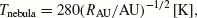(1)(2)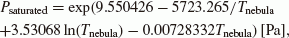(3)

respectively, where RAU is the distance from the Sun,(see Table 1),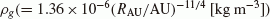is the gas density in the minimum-mass solar nebula,is the Boltzmann constant,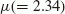is the mean molecular weight andis the mass of the H atom. If the ratio of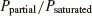exceeds, say, one hundred, most H2O molecules would condense; such a temperature in the solar nebula is 130 K. Most of the CO molecules would be in the gas phase for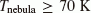(Aikawa et al., 1999). The minimum temperature for carbon oxides to avoid condensation is assumed to be 70 K. We consider several values of the initial temperature of icy planetesimals between 130 K and 70 K. These values correspond to 4 AU and 16 AU, respectively, in the solar nebula (Eq. (1)). We also assume that all condensed H2O is crystalline ice.

We take several values between 10 km and 1000 km for the planetesimals’ radius (Johansen et al., 2007; Cuzzi et al, 2008; Chambers, 2010). The maximum value, 1000 km, is obtained by Johansen et al (2007) by the numerical simulation of planetesimal formation due to gravitational instability, assuming that the magneto-rotational instability is active. Although the formation time of planetesimals is still unknown, we assume planetesimals are formed instantaneously when the condition for the formation of planetes-imals is satisfied at an orbital radius in the solar nebula. We assume a planetesimal has a constant radius and we do not take into account the growth of a planetesimal. If we consider the growth of a planetesimal, the amount of radionu-clide on the additional surface due to the growth would be lower than those in early-formed internal parts. This might lead to a lower temperature evolution on the surface, but this would not affect the center of the planetesimal as long as it is large enough. Compression due to gravity might be significant with a radius larger than 500 km (see p. 247 of Lewis, 1997). We omit the effects of gravitational compression for simplicity.

The main heat source is the decay energy of the shortlived nuclide 26Al, the half-life of which is 0.72 Myr. Another short-lived nuclide, 60Fe could also be a heat source for the planetesimals (e.g. Ito and Ganguly, 2004). We will discuss the effect of 60Fe in Section 4.4. The highest initial ratio of 26Al/27 Al in the solar system would be 5.0 × 10-5, the canonical value of Ca-Al-rich inclusions (CAIs) (e.g. MacPherson et al., 1995). CAIs are thought to be the earliest solids in the Solar System (4567 Ma Amelin et al., 2010). We take several values between 5.0 × 10-5 and 8.0 × 10-7 for the initial ratio of 26Al/27Al. We take the upper limit to be equal to the canonical value of CAI, which means a planetesimal formed just after the formation of the solar nebula. We choose the lower limit because the temperature does not reach the melting point of ice if the initial ratio is smaller than that. These values correspond to the time of the CAI formation and 4.3 Myr after the CAI formation, respectively.

### 2.2 Basic equations

We use the heat-conduction equation for a spherically-symmetric body to calculate the temperature evolutions. The equation is written as follows,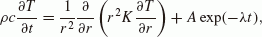(4)

whereis the density, c is the specific heat, T is the temperature, t is time, r is the radius from the center, K is the thermal conductivity, A is the initial radiogenic-heat-generation rate per unit volume, andis the decay constant of a radionuclide. The initial condition (at) iswhere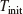is the initial temperature, for which we take two values: 130 K and 70 K (see Section 2.1). The boundary condition at the centerisand the boundary condition at the surfaceis assumed to be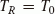, i.e., the planetesimal has a uniform temperature identical to the surrounding nebula. When the melting of ice and the chemical reactions (described in Section 2.4) take place, the latent heat of ice melting and the chemical-reaction heats are taken into account. We solved Eq. (4) using a finite difference method, and an explicit method of time integration is used (see Section 2.5, where we discuss the time steps and the stability). The density, specific heat and thermal conductivity of each material (ice, water and rocks) are shown in Table 2, and all of these values are assumed to be constant. We assume that water and ice have the same density, for simplicity, and the values for rocks are based on Yomogida and Matsui (1983) for Leoville (CV3), and the values for ice and water are based on Murphy and Koop (2005) and Chronological Scientific Tables (2010). We use the mass-weighted mean specific heat, the volume mean density and thermal conductivity for a region where several materials are mixed. For example, an initial icy planetesimal contains ice and rocks (see Section 2.3), and the mean values are given by,(5)(6)

and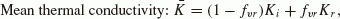(7)

whererepresents the mass ratio of rocks to the total,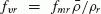represents the volume ratio of rocks to the total, the symbol “ -” denotes the mean value, and the subscripts i and r denote ice and rocks, respectively. The values obtained areandfor the values of the parameters in Table 2.

The only radionuclide considered in this work is 26Al, the decay constantof which is 9.63 x 10-7 year-1.We take the thermal energy per26 Al decay (Edecay) as 3.16 MeV (Schramm et al., 1970), and the abundance of Al () from the CI carbonaceous chondrite (Lodders and Fegley, 1998). The initial heat-generation rate per unit volume is given by,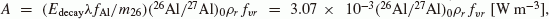where m26 is the mass of an26 Al nucleus. Note that the initial heat generation rate per unit volume depends on the initial ratio of 26Al/27Al, which we choose to be in the range 5.0 x 10-5 to 8.0 x 10-7 (see Section 2.1).

### 2.3 Core formation model of icy planetesimals

An initial icy planetesimal is made of ice and rocks; the latter would be small grains (≥ 1mm), chondrules and CAIs embedded among the ice. The melting temperature, Tmelt, of the ice depends on the pressure, but we assume that the melting temperature of the ice is 273 K, for simplicity. The latent heat of the ice melting is 3.34 x 105 J kg-1 (Chronological Scientific Tables, 2010). The internal ice of a planetesimal melts due to the radiogenic heat of26 Al, and rocks would sink toward the center. The planetesimal would then have a structure with a rocky core, a water mantle, an ice melting region and a frozen crust (see Fig. 1).

It is a complicated problem to solve the convective motion of the mixture of rocks, water and ice and settling of rocks. We make an assumption that the rocks begin to settle after the ice is completely melted, and that an icy planetesimal has an initial mean densityduring the settling, i.e.. The mass of a rock m is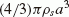, whereis mean material density of the rock and a is the radius of the rock. If the drag force is given by the Stokes formula, the equation of motion for a settling rock in water is given as: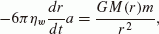(8)

whereis the viscosity of water, and G is the gravitational constant. Substituting the above expressions into Eq. (8), we obtain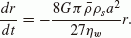(9)

The solution is given by, where r0 is the initial radius at which the rock begins to settle andis the settling time: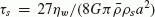. From the above,, for 1-mm-size rocks, is 7 × 106 s. Note this time scale decreases if we take into account the increase in density of the icy planetesimals due to the settling, instead of usingin the expression of M(r) . In the following, we assume that a rocky core, and a water mantle, form instantaneously after the complete melting of ice, becauseis much shorter than the thermal evolution time scale of the order of 1 Myr. The heat flux from the rocky core only raises the temperature of the water mantle by, at most, several degrees above 273 K. Because the increase of the water temperature is small, we simply assume that the temperature of the water mantle is kept equal to 273 K. The region where the temperature could not reach Tmelt remains frozen with the initial composition, which we call the frozen crust.

### 2.4 Chemical reactions

As for the chemical reactions between the rocks and the water, the aqueous alteration and the dehydration reaction are considered. We assume the initial rocks are made of olivine (Mg0.5,Fe0.5)2SiO4 only, for simplicity (see Section 2.1). When ice melts due to 26Al heating, olivine and water react, and aqueous alteration takes place. In our model, we assume the rocky core and the water mantle form after complete ice melting, and that rocks and water could not coexist after the core formation. The ice melting region is the only region where the rocks and water could coexist, and the temperature of the ice melting regions is 273 K. The aqueous alteration occurs during a period of 15 days at 200°C (473 K) and 60 days at 150°C (423 K) (Jones and Brearley, 2006). We obtained the duration of the aqueous alteration at 273 K, calculating the activation temperature Tact based on their results. The reaction-rate coefficient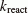is assumed to be given by. The ratio of product to the original reactantduring the reaction time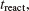is written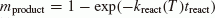. We found that. By using these relations, we find that aqueous alteration occurs during a period of 210 yr at 273 K; this period is very short compared to the thermal evolution time scale (of the order of 1 Myr). We assume that aqueous alteration takes place at 273 K in the ice melting regions.

Serpentine, saponite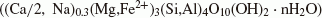and magnetite (Fe3O4) in CI chondrite are the main products due to aqueous alteration (Tomeoka and Buseck, 1988). In this study, we take into account only one chemical-reaction formula for the aqueous alteration:The values of the iron ratio, Fe/(Mg+Fe) of serpentine and talc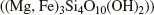are determined based on Tomeoka and Buseck (1988).

We assume the dehydration reaction takes place at 873 K, based on experimental results (Nozaki et al , 2006). When the temperature reaches 873 K in the rocky core, serpentine and talc would be dehydrated, and dehydrated olivine, silica and water would be produced; on the other hand, magnetite could remain at 873 K. We make the assumption that the value of the iron ratio of dehydrated olivine is the same as serpentine and talc, for simplicity. The chemical reaction formula could be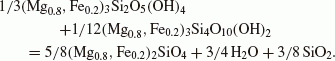H2O is produced in the dehydration reaction; however, the mass ratio of H2O/rocks is small (about 0.07). The effect of water after the dehydration reaction is not considered in this work. The products and reactants of rocks are summarized in Fig. 2. Due to the aqueous alteration, the initial olivine alters into serpentine, talc and magnetite. The dehydrated olivine and silica are produced from serpentine and talc by the dehydration reaction. If the temperature decreases after the dehydration reaction, which releases water around the dehydrated rocks, the aqueous alteration may occur again. However, for simplicity, we do not take this reaction into account.

The chemical-reaction heats are included in the calculation and those are calculated from the enthalpy of each mineral. We take the enthalpies of minerals from Holland and Powell (1998). Calculated results show that the aqueous alteration is an exothermic reaction and its heat is 2. 77 × 105 Jkg-1, and the dehydration reaction is an endothermic reaction and its heat is 4.17 × 105 J kg-1. The dehydration reaction needs more heat than aqueous alteration produces.

The thermal effect of the aqueous alteration is described as follows. From the formula of the aqueous alteration, we found that water and an anhydrous mineral (olivine) are consumed with a mass ratio of 0.1. As a result, the mass of the hydrous minerals (serpentine, saponite and magnetite) is 1.1 times as large as the mass of the original anhydrous mineral. The heat production due to the aqueous alteration is nine times larger than the latent heat of the ice melting required to supply the water consumed by the aqueous alteration. Once the ice begins to melt and a small amount of water is generated, the aqueous alteration which takes place produces enough heat to melt more ice which, in turn, causes further aqueous alteration. Hence, once the ice begins to melt, all the anhydrous minerals are converted to hydrous minerals instantaneously. The heat produced by the aqueous-alteration reaction melts ice of mass nine times as large as the mass of water consumed by the aqueous alteration, as mentioned above. Therefore, just after the instantaneous reaction, water appears. The mass of the water appears after the aqueous alteration is eight times as large as the mass of water consumed in the aqueous-alteration reaction.

### 2.5 Numerical methods

We solved the basic equation (Eq. (4)) as follows. Hereafter we write, which represents the time at the n-th step and position of j-th mesh point. The first term of the RHS of Eq. (4) is approximated by using a finite-difference method with. We have(10)

for, and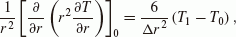(11)

for j = 0. Equation (11) is derived as follows. The Taylor expansion of T r around r = 0is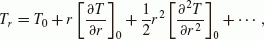(12)

and the limit ofleads(13)

On the other hand, we have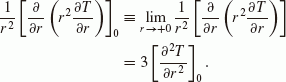(14)

From Eqs. (13) and (14), we find Eq. (11).

In order to perform the time integration, we use the explicit method. From Eq. (4), for, we can write(15)

From Eqs. (4) and (11), for j = 0, we can write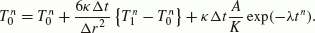(16)

The analytic solution of Eq. (4) is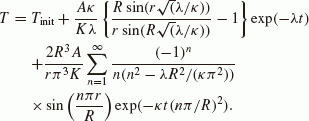(17)

Equation (17) is the temperature profile of bodies with the Dirichlet boundary condition (Carslaw and Jaeger, 1959).

We compare our numerical solution to the analytic solution using the errordefined by(18)

For the stability of numerical simulations, the Courant-Friedrichs-Lewy conditions should be fulfilled. The CFL condition is given by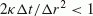. We examined our numerical code, which satisfies the CFL condition, for a 500-km-size rocky planetesimal. As seen from Figs. 3 and 4, the solution obtained by our numerical code is in good agreement with the analytic solution. We usedfor all our numerical simulations shown in Section 3 (e.g., for planetesimals with a radius of 500 km,yr and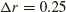km).

When the temperature T becomes slightly above the melting temperature of ice,, as a result of numerical integration, the ice partially melts and is converted to water, keeping the temperature equal to Tmelt. Then, the aqueous-alteration reaction begins to occur, and the reaction proceeds as a sort of chain reaction (see Section 2.4). Numerically, the following changes take place at the time step at which T exceeds Tmelt: (1) All the anhydrous rocks are converted to hydrous rocks. The mass of the hydrous rocks is 1.1 times as large as the original anhydrous rocks, i.e.,whereis the mass of the hydrous rocks among a unit mass of the mixture of hydrous rocks, ice and water. (2) The mass of ice is reduced by 0.9 times the mass of the original anhydrous rocks, i.e.,whereis the mass of ice among a unit mass of the mixture (ice, rock and water). (3) The mass of water is 0.8 times the mass of the original anhydrous rocks, i.e.,, whereis the mass of water among a unit mass of the mixture. Note that before aqueous alteration,, and after aqueous alteration,, and the law of mass conservation is satisfied.

After the completion of the aqueous alteration, the remaining ice begins to melt to form water without being consumed by the aqueous-alteration reaction, keeping the temperature equal to Tmelt. During this stage, the changes ofandin one time step are given by(19)

Whenreaches 0, the ice has totally melted. Then, the hydrous rocks are assumed to fall onto the central rocky core instantaneously. In the rocky core, we solve Eq. (4) by using parameters for rocks instead of mean values of the ice and rock mixture.

When the rocky core reaches the temperature for the dehydration reaction,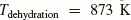, we calculate the change of the mass of hydrated rock in one time step by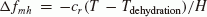, where H is the en-dothermic heat for the dehydration reaction, keeping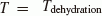(see Section 2.4). When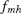reaches 0.3, the serpentine and talc are totally dehydrated. On the other hand, magnetite, the mass ratio of which is 0.3, remains unchanged at 873 K (see Section 2.4). After that, we solve Eq. (4) by using parameters for rocks again.

At the lower boundary of the frozen crust, which contacts with the water mantle, the heat flux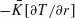in the frozen crust is supplied by the latent heat of water condensation in the water mantle. The thickness of water which condenses during a time stepjust below the boundary is given by. Becausein our calculation, the fraction of the partial freezing is memorized and used in the calculation in the next time step. In the case where the water mantle contacts the ice melting region, a calculation similar to the freezing of the water mantle is carried out including the heating by 26Al simultaneously.

Although there is the heat flux from the inside of the rocky core at the outer boundary of the rocky core, the temperature change in the water mantle due to the flux is estimated to be, at most, several degrees of kelvin, and we just assume that the temperature of the water mantle is kept equal to 273 K.

## 3. Results

We solved Eq. (4) by numerical simulation; the results are shown in the following. The used model parameters of numerical simulations, and brief results, are shown in Tables 3 and 4, whereis the time after CAI formation in Myr, tice is the duration of ice melting in Myr, tdehydration is the duration of the dehydration reaction in Myr, and Tpeak is the peak temperature at the center in kelvin. As seen from Tables 3 and 4, we find that icy planetesimals formed 2.4 Myr after CAI formation could not reach the melting temperature of ice. In these models, it is required that icy planetesimals are formed within 2.4 Myr after CAI formation to melt the ice.

Figure 5 shows the temperature evolutions at the centers of different-sized icy planetesimals with radii 10 km, 50 km, 100 km, 500 km and 1000 km which formed 1.0 Myr after CAI formation with 130 K for the initial temperature (models 10–10A, 50–10A, 100–10A, 500–10A and 1000–10A, respectively). Icy planetesimals over 50 km in radius experience ice melting, aqueous alteration and the dehydration reaction. The duration of ice melting beginning at 1.3 Myr and ending at 1.5 Myr after CAI formation, and the dehy-dration reaction beginning at 2.3 Myr and ending at 3.3 Myr after CAI formation are the same for icy planetesimals having radii over 50 km. This means that thermal conduction is not of effect for these sizes. By way of contrast, the 10-km-size icy planetesimal (model 10–10A) could not attain the melting temperature of ice. Thus, thermal conduction is needed for a 10-km-size icy planetesimal formed within 0.5 Myr after CAI formation to reach the melting temperature of ice (see Tables 3 and 4).

### 3.2 Initial temperature

Temperature evolutions at the centers of different-sized icy planetesimals, with radii 10 km, 50 km, 100 km, 500 km and 1000 km, which formed 1.0 Myr after CAI formation with 70 K for the initial temperature (models 10–10B, 50-10B, 100–10B, 500–10B and 1000–10B, respectively), are shown in Fig. 6. Compared to Fig. 5, only the initial temperature is different. Icy planetesimals over 50 km show a dehydration reaction, but could not exceed the dehydration temperature (873 K). The timing and duration of ice melting are also different, because the energy, which is required to reach the ice melting temperature and dehydration temperature, for low initial temperature models (70 K) is larger than that for high temperature models (130 K). Comparing the results between Tables 3 and 4, we note that the initial temperature also affects the starting point and the duration of melting and dehydration reaction.

### 3.3 Formation time

The results for 500-km-size icy planetesimals, which formed 1.0 Myr, 1.5 Myr, 2.0 Myr and 2.4 Myr after CAI formation with 130 K for the initial temperature (models 500–10A, 500–15A, 500–20A and 500–24A, respectively), are shown in Fig. 7. The formation time (i.e. the initial ratio of 26Al/27Al) largely affects the temperature evolution, i.e. the occurrence of the ice melting, the aqueous alteration and the dehydration reaction, as well as the beginning and the duration of chemical reactions.

The details of an icy planetesimal having a 500-km radius, and 130 K for the initial temperature, formed at 1.0 Myr after CAI formation (model 500–10A), are shown in Figs. 8 to 11. Before the ice melting begins (e.g., 1.2 Myr in Fig. 9), the temperature is almost constant with radius, with the exception of a thin surface region. After the ice melting, there are two regions where the temperature is almost constant, i.e. the rocky core except for a thin surface region of the rocky core, and the water mantle; on the other hand, the frozen crust has a larger temperature gradient (see Fig. 9). A rocky core and a water mantle are formed at 1.5 Myr after CAI formation. The radii of the rocky core (270 km) and the thickness of the water mantle (220 km) are almost the same over 10 Myr (Fig. 8).

Figure 10 shows the mass ratio of ice, water and rocks at 6.0 Myr after CAI formation. Due to the dehydration reaction, the formation of dehydrated rocks (olivine and silica) occurs in the rocky core (260 km) except for a thin outer shell (10 km). Cumulative energies of heat sources (radiogenic heat and heat of aqueous alteration) and heat consumptions (change in internal energy, latent heat of ice, heat of dehydration reaction and radiation at the surface) are shown in Fig. 11. The heat of the aqueous alteration is released in a short period of 0.007 Myr around 1.3 Myr after CAI formation. On the other hand, the consumption of heat by the dehydration reaction takes longer than the aqueous alteration (2.3 Myr-3.3 Myr after CAI formation). The total energy (difference between heat source and heat consumption) is kept almost equal to zero, which means that the energy conservation is well satisfied in the numerical simulation.

## 4. Discussions

### 4.1 Rocky core

The temperature evolutions at the centers of icy planetes-imals, having radii over 50 km, after the dehydration reaction are different for different radii (Fig. 5). We examine the radii of rocky cores to see the cause of this difference. The radii of rocky cores of icy planetesimals for 50 km, 100 km, 500 km and 1000 km (models 50–10A, 100–10A, 500–10A and 1000–10A, respectively) are 25 km, 53 km, 270 km and 550 km, respectively. It is expected that the temperature evolution in a rocky core would evolve in the same way as a rocky planetesimal, which has a radius of rocky core, formed 1.5 Myr after CAI formation (i.e. the time when the rocky core formed) with 273 K for the initial temperature. The temperature evolutions at the center of rocky planetesimals are shown in Fig. 12. The temperature evolutions at the centers of an icy planetesimal, and that of a rocky planetesimal, are nearly the same provided that the radius of the rocky core of the icy planetesimal is the same as the radius of the rocky planetesimal. The difference in size determines the rocky core’s radius as long as the time of formation is the same, and the radius of the rocky core, in turn, determines the temperature evolution of the rocky core.

### 4.2 The effect of chemical reactions

In order to elucidate the effects of chemical reactions (the aqueous alteration and the dehydration reaction), we carried out a numerical simulation of temperature evolution without chemical reactions. We used the same parameters of model 500–10A (500-km-size icy planetesimal formed 1.0 Myr after CAI formation with 130 K for the initial temperature). We compare these results with, and without, chemical reactions in Fig. 13. As seen from Fig. 13, the ice melting begins at 1.3 Myr and ends at 1.8 Myr after CAI formation; this period is longer than model 500–10A with the aqueous alteration. Aqueous alteration plays an important role in ice melting (Cohen and Coker, 2000).

### 4.3 Effect of porosity

Meteorites are composed of porous material (Yomogida and Matsui, 1983). The parent body of meteorites (planetesimals or asteroids) would also be porous. It is, therefore, worthwhile considering the effect of porosity. Thermal conductivity is reduced in porous material because of the small contact areas of the grains (Haruyama et al , 1993). However, a porous material enables vapor flow before a melting temperature is achieved, and this contributes to heat transfer. It is difficult to determine whether the effective thermal conductivity decreases or increases.

Here, we simply calculate two cases, in which the thermal conductivity is twice and a half, respectively, our standard value (see Section 2.2), in order to gauge the effect of the change of thermal conductivity in the frozen crust. We assume the pores are filled with water after the melting of ice. The effect of porosity will appear before ice melting and does not show after the melting of ice starts. Figure 14 shows the result of icy planetesimals having a 50-km radius and 130 K for the initial temperature formed 1.0 Myr after CAI formation (model 50–10A) with twice, half of, and just equal to, the standard value for the thermal conductivity in the frozen crust. The ice melting region changes as a result of the effect of porosity. The radius of the rocky core and the thickness of the water mantle in an icy planetesimal are greater when we halve the thermal conductivity, but smaller when we double the thermal conductivity. However, the beginning, and the duration, of ice melting does not change. This means that the effect of porosity in the frozen crust does not affect the timing and duration of these events. If a rocky core is porous, the core would contain water in the pores; in this case, the hydrothermal reaction might continue after the core formation. We leave this issue for future work.

### 4.4 60 Fe as a heat source

Some authors take into account both26 Al and 60Fe as heat sources for the parent bodies of meteorites. Ito and Ganguly (2004) used a Solar System initial ratio of 60Fe/56Fe 3 × 10−7 (Tachibana and Huss, 2003). Recent studies show a Solar System initial ratio of 60Fe/56Fe < 1 × 10-8 (Spivak-Brindorf et al., 2011; Tang and Dauphas, 2011) and < 2 × 10−7 (Telus et al., 2011). It is worthwhile considering the effects of 60Fe using recent values of the initial ratio of 60Fe/56Fe.

The heat production rate of 26Al and 60Fe is shown in Fig. 15. At first, the heat production rate of26Al is larger than that of 60Fe. But the heat production rate of 60Fe exceeds that of26 Al at 7 Myr for 60Fe/56Fe = 2.0 × 10−7, and at 11 Myr for 60Fe/56Fe = 1.0 × 10−8. This is because 60Fe has a longer half-life than26Al (0.72 Myr; see Section 2.1). The half-life of 60Fe is 2.6 Myr (Rugel et al., 2009).

We have calculated the thermal evolution of icy plan-etesimals with 26Al and 60Fe as heat sources. We take the thermal energy released by the decay of one 60Fe nucleus to be 2.71 MeV (Tuli, 2003), and the abundance of Fe from CI carbonaceous chondrite (Lodders and Fegley, 1998). Figure 16 shows the result for icy planetesimals having radii of 100 km and 50 km and 130 K for the initial temperature formed 1.0 Myr after CAI formation (models 100–10A and 50–10A) for three values of 60Fe/56Fe: 1.5 × 10−7,0.8 × 10−8 and 0 (without 60Fe). The values of 60Fe/56Fe at 1.0 Myr after CAI formation are calculated from the Solar System initial ratio of 60Fe/56Fe (2.0 × 10−7 and 1.0 × 10−8). In these calculations, we include aluminum heating with 26Al/27Al = 2× 10−5 at 1.0 Myr after CAI formation. The early evolution before the beginning of the dehydration reaction is not affected by the abundance of 60Fe, because 26Al heating prevails, as shown in Fig. 15. In the results for icy planetesimals with 60Fe/56Fe = 1.5 × 10−7, the peak temperature is higher and the duration of the dehydration reaction is shorter than in the results for icy plan-etesimals without 60Fe. However, the temperature profile does not change in the results for icy planetesimals with 60Fe/56Fe = 0.8 × 10−8. A large Solar System initial ratio of 60Fe/56Fe is needed to affect the temperature evolution of icy planetesimals. Further studies on the initial ratio of 60Fe/56Fe are needed in order to consider 60Fe as a heat source.

### 4.5 Comparison of the results to the chronological data of meteorites

The planetesimals with H2O ice, but without CO ice, are located at 4 AU-16 AU in the early solar nebula, where most of the water molecules condense, and have an ice/rock mass ratio, of 1.72 (see Section 2.1). It is not well known if there exist meteorites which originate from such icy plan-etesimals.

We have compared the timing of aqueous alteration in carbonaceous chondrite with our results. The timing of aqueous alteration is obtained by 53Mn-53Cr age studies. The half-life of 53Mn is 3.7 Myr. From recent isotopic studies for aqueously-altered carbonaceous chondrites, the initial ratios of 53Mn/55Mn are given as (2.3 ± 0.1) × 10−6 for Mokoia (CV3) (Hutcheon et al, 1998), (2.7 ± 1.0) × 10−6 for Allende (CV3) (Trinquier et al, 2008), (2.3 ± 0.5) × 10−6 for Vigarano (CV3) (Jogo et al, 2009), (2.7 ± 0.8) × 10−6 for Murchison (CM2.5), and (2.8 ± 0.4) × 10−6 for ALH 83100 (CM2.1) (Fujiya et al, 2010). The initial ratio of 53Mn/55Mn of CAIs is (9.1 ± 1.7) × 10−6 and the estimated absolute age of these CAIs is 4568 Ma (Nyquist et al , 2009). If we use these CAIs as a time marker, aqueous alteration occurred at 6 Myr-7 Myr after CAIs. Our calculation shows that icy planetesimals formed 2.0 Myr after CAI formation at 4 AU having radii over 50 km (models 50– 20A, 100–20A, 500–20A and 1000–20A) satisfy those times of aqueous alteration. However, our model assumes that the initial water/rock mass ratio is equal to 1.72, which corresponds to the solar nebula abundance, and we also assume that all the rocks have once suffered aqueous alteration at 273 K. We should take into account different ice/rock ratios, as well as hydrothermal reactions in porous rock cores, in order to simulate the thermal evolutions of the parent bodies of carbonaceous chondrite.

## 5. Conclusions

The thermal history and time evolution of planetesimals in the early solar nebula is one of the important issues for the elucidation of planet formation. We have performed numerical simulations for the thermal and time evolutions of icy planetesimals to constrain on its size and time of its formation under certain conditions.

Icy planetesimals, which are larger than 50 km in radius and which formed 1.0 Myr after CAI formation with 130 K for an initial temperature, could experience ice melting, aqueous alteration and a dehydration reaction. The duration of the ice melting (1.3–1.5 Myr after CAI formation) and the dehydration reaction (2.3–3.3 Myr after CAI formation) are the same for icy planetesimals having radii greater than 50 km. Thermal conduction is not of effect for these sizes. The radius of the rocky core depends on the icy plan-etesimal radius, which, in turn, affects the temperature evolution of the rocky core. On the other hand, an icy planetesi-mal 10 km in radius, formed at 1.0 Myr after CAI formation with 130 K for the initial temperature, could not attain the melting temperature of the ice. Icy planetesimals formed at 2.4 Myr after CAI formation, or later, could not attain the melting temperature of the ice either, regardless of their size and initial temperature.

The beginning, and the duration, of the ice melting and the chemical reactions are the same for different sizes of icy planetesimals formed at the same time, as long as their radius is greater than 50 km, but are different for the same-size icy planetesimals formed at different times. The difference in the formation time of an icy planetesimal is the most influential parameter for the occurrence of ice melting, aqueous alteration and the dehydration reaction, as well as the beginning, and duration, of chemical reactions. The effects of chemical reactions are also considered. Aqueous alteration has the effect to melt ice quickly.

The growth of icy planetesimals, the differentiation of the rocky core, and the hydrothermal reaction due to water contained in the pores of the rocky core, are aspects which should be considered in future work.

## References

1. Aikawa, Y., T. Umebayashi, T. Nakano, and S. M. Miyama, Evolution of molecular abundances in proto-planetary disks with accretion flow, Astrophys. J., 519, 705–725, 1999.

2. Amelin, Y., A. Kaltenbach, T. Iizuka, C. H. Stirling, T. R. Ireland, M. Petaev, and S. B. Jacobsen, Importance of uranium isotope variations for chronology of the Solar System’s first solids, Lunar Planet. Sci. Conf., 41, #1648, 2010.

3. Anders, E. and N. Grevesse, Abundances of the elements: Meteoritic and solar, Geochim. Cosmochim. Acta, 53, 197–214, 1989.

4. Bland, P. A., M. D. Jackson, R. F. Coker, B. A. Cohen, J. B. W. Webber, M. R. Lee, C. M. Duffy, R. J. Chater, M. G. Ardakani, D. S. McPhail, D. W. McComb, and G. K. Benedix, Why aqueous alteration in asteroids was isochemical: High porosity ≠ high permeability, Earth Planet. Sci. Lett., 287, 559–568, 2009.

5. Carslaw, H. S. and J. C. Jaeger, Conduction of Heat in Solids, Oxford Univ. Press, New York, 1959.

6. Chambers, J. E., Planetesimal formation by turbulent concentration, Icarus, 208,505–517, 2010.

7. Chronological Scientific Tables, edited by National Astronomical Observatory of Japan, Maruzen Co., Ltd. 2010 (in Japanese).

8. Cohen, B. A. and R. F. Coker, Modeling of liquid water on CM meteorite parent bodies and implications for amino acid racemization, Icarus, 145, 369–381,2000.

9. Crovisier, J., Physics and chemistry of comets: recent results from comets Hyakutake and Hale-Bopp Answers to old questions and new enigmas, Faraday Discuss., 109, 437–452, 1998.

10. Cuzzi, J. N., R. C. Hogan, and K. Shariff, Toward planetesimals: dense chondrule clumps in the protoplanetary nebula, Astrophys. J., 687, 1432–1447, 2008.

11. Desch, S. J., J. C. Cook, T. C. Doggett, and S. B. Porter, Thermal evolution of Kuiper belt objects, with implications for cryovolcanism, Icarus, 202, 694–714, 2009.

12. Fujiya, W., N. Sugiura, K. Ichimura, N. Takahata, and Y. Sano, Mn-Cr ages of carbonates in Murchison and ALH 83100 CM chondrites, Lunar Planet. Sci. Conf., 41, #1644, 2010.

13. Ghosh, A. and H. Y. McSween, Jr., A thermal model for the differentiation of asteroid 4 Vesta, based on radiogenic heating, Icarus, 134, 187–206, 1998.

14. Grady, M. M., A. B. Verchovsky, I. A. Franchi, I. P. Wright, and C. T. Pillinger, Light element geochemistry of the Tagish Lake CI2 chondrite: Comparison with CI1 and CM2 meteorites, Meteorit. Planet. Sci., 37, 713–735,2002.

15. Grimm, R. E. and H. Y. McSween, Jr., Water and the thermal evolution of carbonaceous chondrite parent bodies, Icarus, 82, 244–280, 1989.

16. Haruyama, J., T. Yamamoto, H. Mizutani, and J. M. Greenberg, Thermal history of comets during residence in the Oort cloud: Effect of radiogenic heating in combinatinon with the very low thermal conductivity of amorphous ice, J. Geophys. Res., 98, 15079–15090, 1993.

17. Hayashi, C., Structure of the solar nebula, growth and decay of magnetic fields and effects of magnetic and turbulent viscosities on the nebula, Progr. Theor. Phys. Suppl., 70, 35–53, 1981.

18. Holland, T. J. B. and R. Powell, An internally consistent thermodynamic data set for phases of petrological interest, J. Metamorph. Geol., 16, 309–343, 1998.

19. Hutcheon, I. D., A. N. Krot, K. Keil, D. L. Phinney, and E. R. D. Scottt, 53Mn-53Cr dating of fayalite formation in the CV3 chondrite Mokoia: Evidence for asteroidal alteration, Science, 282, 1865–1867, 1998.

20. Ito, M. and J. Ganguly, Potassium diffusion in melilite: Experimental studies and constraints on the thermal history and size of planetesimals hosting CAIs, Meteorit. Planet. Sci., 39, 1911–1919, 2004.

21. Jogo, K., T. Nakamura, T. Noguchi, and M. Y. Zolotov, Fayalite in the Vigarano CV3 carbonaceous chondrite: Occurrences, formation age and conditions, Earth Planet. Sci. Lett., 287, 320–328, 2009.

22. Johansen, A., J. S. Oishi, M.-M. Mac Low, H. Klahr, T. Henning, and A. Youdin, Rapid planetesimal formation in turbulent circumstellar disks, Nature, 448, 1022–1025, 2007.

23. Johansen, A., A. Youdin, and M.-M. Mac Low, Particle clumping and planetesimal formation depend strongly on metallicity, Astrophys. J., 704, L75–L79, 2009.

24. Jones, C. L. and A. J. Brearley, Experimental aqueous alteration of the Allende meteorite under oxidizing conditions: Constraints on asteroidal alteration, Geochim. Cosmochim. Acta, 70, 1040–1058, 2006.

25. LaTourrette, T. and G. J. Wasserburg, Mg diffusion in anorthite: implications for the formation of early solar system planetesimals, Earth Planet. Sci. Lett., 158, 91–108, 1998.

26. Lewis, J. S., Physics and Chemistry of the Solar System, Academic Press, 1997.

27. Lodders, K. and B. Fegley, Jr., The Planetary Scientist’s Companion, Oxford Univ. Press, New York, 1998.

28. MacPherson, G. J., A. M. Davis, and E. K. Zinner, The distribution of aluminum-26 in the early Solar System—A reappraisal, Meteoritics, 30, 365–386, 1995.

29. McSween, H. Y., Jr., Meteorites and Their Parent Planets, Cambridge Univ. Press, New York, 1999.

30. Miyamoto, M., N. Fujii, and H. Takeda, Ordinary chondrite parent body: An internal heating model, Proc. Lunar Planet. Sci., 12B, 1145–1152, 1981.

31. Murphy, D. M. and T. Koop, Review of the vapour pressures of ice and supercooled waterfor atmospheric applications, Q. J. R. Meteorol. Soc., 131, 1539–1565, 2005.

32. Nozaki, W., T. Nakamura, and T. Noguchi, Bulk mineralogical changes of hydrous micrometeorites during heating in the upper atmosphere at temperatures below 1000°C, Meteorit. Planet. Sci., 41, 1095–1114, 2006.

33. Nyquist, L. E., T. Kleine, C.-Y. Shih, and Y. D. Reese, The distribution of short-lived radioisotopes in the early solar system and the chronology of asteroid accretion, differentiation, and secondary mineralization, Geochim. Cosmochim. Acta, 73, 5115–5136, 2009.

34. Palguta, J., G. Schubert, and B. J. Travis, Fluid flow and chemical alteration in carbonaceous chondrite parent bodies, Earth Planet. Sci. Lett., 296, 235–243, 2010.

35. Rugel, G., T. Faestermann, K. Knie, G. Korschinek, M. Poutivsev, D. Schumann, N. Kivel, I. Günther-Leopold, R. Weinreich, and M. Wohlmuther, New measurement of the 60Fe half-life, Phys. Rev. Lett., 103, 072502, 2009.

36. Schramm, D. N., F. Tera, and G. J. Wasserburg, The isotopic abundance of 26Mg and limits on 26Al in the early Solar System, Earth Planet. Sci. Lett., 10, 44–59, 1970.

37. Schubert, G., J. D. Anderson, B. J. Travis, and J. Palguta, Enceladus: Present internal structure and differentiation by early and long-term radiogenic heating, Icarus, 188, 345–355, 2007.

38. Spivak-Brindorf, L. J., M. Wadhwa, and P. E. Janney, 60Fe-60Ni chronology of the D’Orbigny angrite: Implications for the initial solar system abundance of 60Fe, Lunar Planet. Sci. Conf., 42, 2281, 2011.

39. Tachibana, S. and G. Huss, The initial abundance of 60Fe in the Solar System, Astrophys. J., 588, L41–L44, 2003.

40. Tang, H. and N. Dauphas, Constraints from achondrites on the initial 60Fe/56Fe ratio of the solar system, Lunar Planet. Sci. Conf., 42, 1068, 2011.

41. Telus, M., G. R. Huss, K. Nagashima, R. C. Ogliore, S. Tachibana, and C. E. Jilly, Possible heterogeneity of 60Fe in chondrules from primitive ordinary chondrites, Lunar Planet. Sci. Conf., 42, 2559, 2011.

42. Tomeoka, K. and P. R. Buseck, Matrix mineralogy of the Orgueil CI carbonaceous chondrite, Geochim. Cosmochim. Acta, 52, 1627–1640, 1988.

43. Travis, B. J. and G. Schubert, Hydrothermal convection in carbonaceous chondrite parent bodies, Earth Planet. Sci. Lett., 240, 234–250, 2005.

44. Trinquier, A., J.-L. Brick, C. J. Allègre, C. Göpel, and D. Ulfbeck, 53Mn-53Cr systematics of the early Solar System revisited, Geochim. Cos-mochim. Acta, 72, 5146–5163, 2008.

45. Tuli, J. K., Nuclear Data Sheets, 100, 347–481, 2003.

46. Turcotte, D. L. and G. Schubert, Geodynamics, Cambridge Univ. Press, New York, 2002.

47. Urey, H. C., The cosmic abundances of potassium, uranium, and thorium and the heat balances of the Earth, the Moon and Mars, Proc. Natl. Acad. Sci., 41, 127–144, 1955.

48. Yamoto, F., Possibility of planetesimal formation due to enhancement of local dust density in the solar nebula, Master Thesis, 2003 (in Japanese).

49. Yomogida, K. and T. Matsui, Physical properties of ordinary chondrites, J. Geophys. Res., 88, 9513–9533, 1983.

50. Youdin, A. N. and J. Goodman, Streaming instabilities in protoplanetary disks, Astrophys. J., 620, 459–469, 2005.

51. Young, E. D., The hydrology of carbonaceous chondrite parent bodies and the evolution of planet progenitors, Phil. Trans. R. Soc. Lond., 359, 2095–2110, 2001.

52. Young, E. D., R. D. Ash, P. England, and D. Rumble III, Fluid flow in chondritic parent bodies: Deciphering the compositions of planetesi-mals, Science, 286, 1331–1335, 1999.

## Acknowledgments

We thank Drs. Tomoki Nakamura, Tomoaki Kubo, Ryuji Okazaki, Hiizu Nakanishi and Saburo Miyahara for their comments and discussions. We also thank Dr. Motoo Ito and an anonymous reviewer for their helpful reviews. Numerical simulations were in part performed on the general-purpose PC farm at the Center for Computational Astrophysics, CfCA, of the National Astronomical Observatory of Japan. This work is supported by Grant-in-Aid for JSPS Fellows (21-2143) from the Ministry of Education, Culture, Sports, Science and Technology.

## Author information

Authors

### Corresponding author

Correspondence to Shigeru Wakita.

## Rights and permissions

Reprints and Permissions

Wakita, S., Sekiya, M. Thermal evolution of icy planetesimals in the solar nebula. Earth Planet Sp 63, 1193–1206 (2011). https://doi.org/10.5047/eps.2011.08.012

• Revised:

• Accepted:

• Published:

• Issue Date:

### Key words

• Icy planetesimals
• thermal histories
• solar nebula